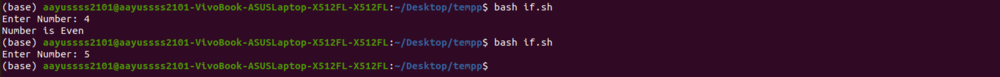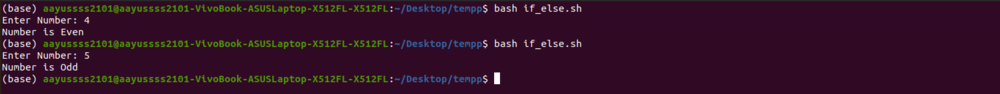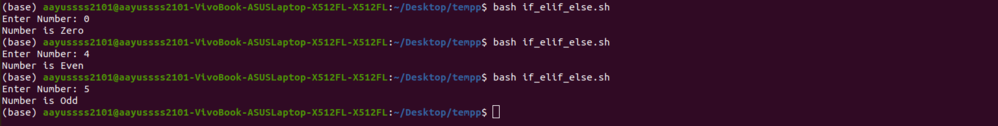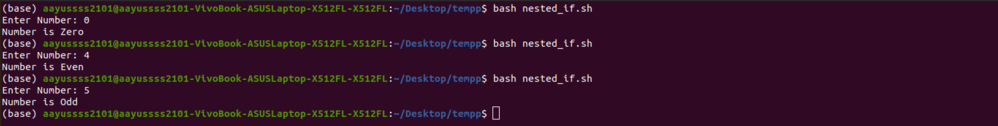GeeksforGeeks App
Open AppBrowser
Continue

# Bash Scripting – If Statement

Bash is an interpreter for command languages. It is a default command interpreter on most GNU/Linux systems and is widely available on various operating systems. The name is an abbreviation for Bourne-Again SHell. Scripting enables for the execution of instructions that would otherwise be executed one by one interactively.

## If Statement

The basic syntax of an if statement is the following:

```if CONDITION
then
COMMANDS
fi```

The if statement is composed of the if keyword, the conditional phrase, and the then keyword. The fi keyword is used at the end of the statement. The COMMANDS gets executed if the CONDITION evaluates to True. Nothing happens if CONDITION returns False; the COMMANDS are ignored.

Example:

The following is an example script that will prompt you to input a number and then it checks whether the given number is even.

```echo -n "Enter Number: "

if [ \$((x%2)) == 0 ]; then
echo "Number is Even"
fi```

Output:Output

## if…else Statement

An if…else sentence has the following basic syntax:

```if CONDITION
then
COMMANDS_1
else
COMMANDS_2
fi```

The COMMANDS_1 will be executed if the CONDITION evaluates to True. Otherwise, COMMANDS_2 will be run if CONDITION returns False. There can only be one else clause in a statement.

Example:

Let’s extend the script in the above example to check if the given number is even or odd.

```echo -n "Enter Number: "

if [ \$((x%2)) == 0 ]; then
echo "Number is Even"
else
echo "Number is Odd"
fi```

Output:Output

## if…elif…else Statement

The following is the basic syntax of an if…elif…else statement:

```if CONDITION_1
then
COMMANDS_1
elif CONDITION_2
then
COMMANDS_2
else
COMMANDS_3
fi```

COMMANDS_1 will be executed if the CONDITION_1 evaluates to True. COMMANDS_2 will be executed if the CONDITION_2 evaluates to True. The COMMANDS_3 is run if none of the test commands evaluate to True.

In the statement, there can be one or more elif clauses. The else clause is optional.

Example:

Let’s augment the code in the above example to add the condition to check for zero.

```echo -n "Enter Number: "

if [ \$x == 0 ]; then
echo "Number is Zero"
elif [ \$((x%2)) == 0 ]; then
echo "Number is Even"
else
echo "Number is Odd"
fi```

Output:Output

## Nested if statement

If statements can be nested within if statements in Bash. Multiple if statements can be nested inside another if statement. Something like this:

```if CONDITION_1
then
if CONDITION_2
then
COMMANDS_1
else
COMMANDS_2
fi
else
COMMANDS_3
fi```

If both CONDITION_1 and CONDITION_2 evaluate to True, COMMANDS_1 will be run. If CONDITION_2 evaluates to False while CONDITION_1 remains True, COMMANDS_2 will be executed. Otherwise, COMMANDS_3 is executed.

Example:

Let’s tweak the code above a little bit to use nested-if.

```echo -n "Enter Number: "

if [ \$((x%2)) == 0 ]; then
if [ \$x == 0 ]; then
echo "Number is Zero"
else
echo "Number is Even"
fi
else
echo "Number is Odd"
fi```

Output:Output

## Some Notes on CONDITION

These are a few things to keep in my mind while writing test conditions in bash.

1. Ensure whitespaces between the brackets and the actual check/comparison statement.

For example, the following will not work.

`if [\$x==0]`

Bash will report an error about a missing ].

2. Always end the line before adding a new keyword, such as “then.”

If, then, else, elif, and fi are all shell keywords, which means they can’t be used on the same line. Put a “;” between the previous statement and the keyword, or start a new line with the keyword.

3. To use many conditions in one statement, use logical operators.

We can use logical AND(&&) or logical OR(||) operators to use multiple conditions.

For example:

```if [[ \$x -ge \$y ]] && [[ \$x -ge \$z ]]; then
echo "x is greatest"
fi```

Here, -ge is a shorthand for greater than or equal to

My Personal Notes arrow_drop_up